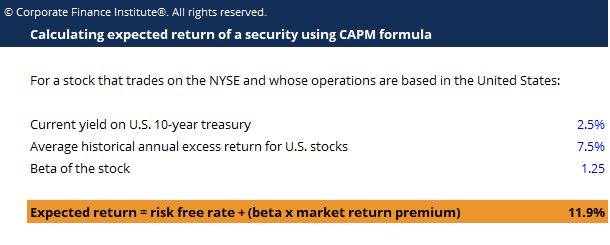CAPM Formula Template

CAPM Formula Template

This CAPM formula template will help you calculate the required rate of return for investing in a security given the risk-free return and risk premium.

This is what the CAPM template looks like:Enter your name and email in the form below and download the free template now!

CAPM Formula Template

The Capital Asset Pricing Model (CAPM) describes the relationship between expected return and risk of investing in a security. It shows that the expected return on a security is equal to the risk-free return plus a risk premium, which is based on the beta of that security.

The formula for calculating CAPM is as follow:

Expected return on a security = Risk-free rate + Beta of the security * (Expected return on market – Risk-free rate)

It is based on the premise that investors have assumptions of systematic risk (also known as market risk or non-diversifiable risk) and need to be compensated for it in the form of a risk premium – an amount of market return greater than the risk-free rate. By investing in a security, investors want a higher return for taking on additional risk.

More Free Templates

For more resources, check out our business templates library to download numerous free Excel modeling, PowerPoint presentation and Word document templates.

• Excel Modeling Templates
• PowerPoint Presentation Templates
• Transaction Document Templates

Financial Analyst Certification

Become a certified Financial Modeling and Valuation Analyst (FMVA)® by completing CFI’s online financial modeling classes and training program!